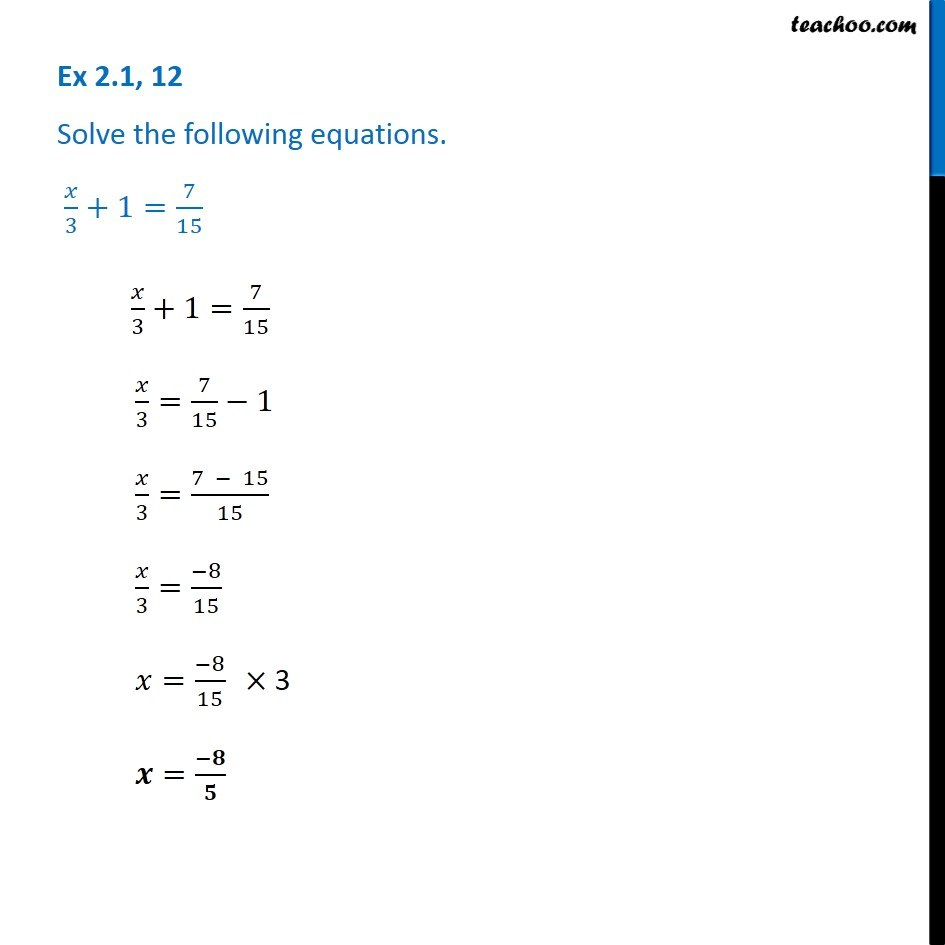Ex 2.1

Chapter 2 Class 8 Linear Equations in One Variable
Serial order wiseGet live Maths 1-on-1 Classs - Class 6 to 12

### Transcript

Ex 2.1, 12 - Chapter 2 Class 8 NCERT - Linear Equations in one variable Solve the following equations. x/3 + 1 = 7/15 x/3 + 1 = 7/15 x/3 = 7/15 - 1 x/3 = (7 - 15)/15 x/3 = (-8)/15 x = (-8)/15 × 3 x = -8/5